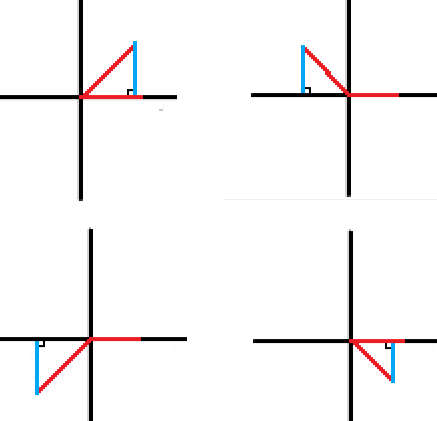How can we help?

### 1 comment

• To form the triangle, draw a line up or down from the terminal side of your reference angle so that the line you draw meets the x-axis at a 90 degree angle. The terminal side of your reference angle will be the hypotenuse of the triangle, and one of the sides will be on the x-axis. In the pictures below, the red is your reference angle and the blue is the line you draw up/down to make the triangle. If you wanted to draw the unit circle around them, you know that the radius of the unit circle = the hypotenuse of the triangle = 1, so the vertex of the triangle that does NOT lie on the x-axis is the one that would lie on the circle.Post is closed for comments.